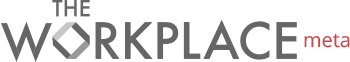## Reputation histogram

4

(click on this box to dismiss)

Generates a histogram of users' reputation. The histogram bins are automatically determined using Doane's formula, and the lowest bin is discarded since it would probably contain so many users that the rest of the histogram would be unreadable. Obviously best viewed as a graph.Q&A about the site for members of the workforce navigating the professional setting

``````DECLARE @BinSize int;

-- After discarding users with < 150 reputation as outliers, calculate
-- an optimal number of histogram bins by applying Doane's Formula.
-- https://en.wikipedia.org/wiki/Histogram#Number_of_bins_and_width
WITH AvidUsers AS (
SELECT Id
, Reputation
FROM Users
WHERE Reputation >= 150
), Mean AS (
SELECT AVG(Reputation) AS μ
FROM AvidUsers
), Moments AS (
SELECT COUNT(Id) AS n
, μ
, STDEV(Reputation) AS σ
, AVG(POWER(CAST(Reputation - μ AS FLOAT), 4)) AS μ4
FROM AvidUsers, Mean
GROUP BY μ
), Kurtosis AS (
SELECT n
, μ4 / POWER(σ, 4) - 3 AS ɣ2
FROM Moments
), DoanesFormula AS (
SELECT CEILING(1 + LOG(n, EXP(1)) + LOG(1 + ɣ2 * SQRT(n / 6), EXP(1))) AS k
FROM Kurtosis
), BinSize AS (
SELECT ROUND(MAX(Reputation) / k, -2) AS BinSize
FROM AvidUsers, DoanesFormula
GROUP BY k
) SELECT @BinSize = BinSize FROM BinSize;

-- Be sure to plot the histogram bins with 0 members as well.
-- e3 is just some arbitrary table with 81 rows.
WITH e1(n) AS ( -- 3
SELECT 1 UNION ALL SELECT 1 UNION ALL SELECT 1
), e2(n) AS ( -- 9
SELECT a.* FROM e1 AS a CROSS JOIN e1 AS b
), e3(n) AS ( -- 81
SELECT a.* FROM e2 AS a CROSS JOIN e2 AS b
), Bins AS (
SELECT @BinSize * (ROW_NUMBER() OVER (ORDER BY n) + 0.5) AS bin
FROM e3
), UserCounts AS (
SELECT (Reputation / @BinSize + 0.5) * @BinSize AS Reputation
, COUNT(Id) AS Count
FROM Users
GROUP BY Reputation / @BinSize
)
SELECT Bins.bin AS Reputation
, COALESCE(Count, 0) AS Count
FROM BinsHold tight while we fetch your results